# 11. Plotting and Displaying Data¶

Plotting and Visualizing data are vital to any scientific analysis package, and Larch provides several methods for data visualization. These are largely built on two types of data display. The first is the line plot (sometimes calld an xy plot), which shows traces of a set of functions y(x). The second type of data display supported is the 2-dimensional image display, in which a grey scale or false color map shows an image representing a 2-dimensional array of intensity.

Though not a dedicated plotting and graphics packages, Larch attempts to provide satisfying graphical displays of data, and the basic plots made with Larch are of high enough quality to include in publications. In addition, both line plots and image display provide interactive features such as zooming in and out, changing properties such as colors and labels. Finally, copying and saving images of the graphics is easy and can be done either with keyboard commands such as Ctrl-C or from dropdown menus on the graphic elements.

## 11.1. Line Plots¶

Larch provides a few functions for making line plots, with the principle function being called plot(). The plot() function takes two arrays: x, the abscissa array, and y, the ordinate array. It also accepts a very large number of optional arguments for setting properties like color, line style, labels, and so on. Most of these properties can also be set after the plot is displayed through the graphical display of the plot itself. The plots are fully interactive so that coordinates can be seen (and written to larch variables) by clicking on the plot, and zooming in on portions of the plot can be done with click-and-drag. Right-clicking will pop up a list of options for zooming out, configuring the plot, or saving a PNG image of the plot. Menus on the window frame give even more options, including Copy-to-clipboard and printing.

Multiple plot windows can be shown simultaneously, each in an independent window, and you can control which one is drawn to with a plot window index win.

### 11.1.1. plot(), newplot(), and oplot()¶

These functions all create a Line Plot from array of x and y data that are taken to be ordered, and so can be drawn as connected by lines from one point to the next. These functions share many options to set labels, colors, and so on to modify the resulting plot. All options are specified by the keyword/value arguments described in the Table of Plot Arguments below.

_plotter.plot(x, y, **kws)
Parameters
• x – array of x values

• y – array of y values – same size as x

• kws – optional commands as listed in Table of Plot Arguments.

Plot y(x) given 1-dimensional x and y arrays – these must be of the same size. Each x, y pair displayed is called a trace.

_plotter.newplot(x, y, **kws)
Parameters
• x – array of x values

• y – array of y values – same size as x

• kws – optional commands as listed in Table of Plot Arguments.

This is essentially the same a plot(), but with the option new=True. The rest of the arguments are as

_plotter.plot(x, y, **kws)
Parameters
• x – array of x values

• y – array of y values – same size as x

• kws – optional commands as listed in Table of Plot Arguments.

This is essentially the same a plot(), but with the option new=False.

Table of Plot Arguments These arguments apply for the plot(), newplot(), and scatterplot() methods. Except where noted, the arguments are available for plot() and newplot(). In addition, the scatterplot() method uses many of the same arguments for the same meaning, as indicated by the right-most column.

argument

type

default

meaning

scatterplot?

title

string

None

Plot title

yes

ylabel

string

None

abscissa label

yes

y2label

string

None

right-hand abscissa label

yes

label

string

None

trace label (defaults to ‘trace N’)

yes

win

integer

1

index of plot window to use (1, 2, …, 16)

yes

side

left/right

left

side for y-axis and label

yes

grid

None/bool

None

to show grid lines

yes

fullbox

bool

True

to show full box (not just left + bottom axes)

no

color

string

blue

color to use for trace

yes

bgcolor

string

white

color to use for plot background

yes

framecolor

string

white

color to use for outer plot frame

yes

gridcolor

string

gray

color to use grid

yes

use_dates

bool

False

to show dates in xlabel (plot() only)

no

linewidth

int

2

linewidth for trace

no

style

string

solid

line-style for trace (solid, dashed, …)

no

drawstyle

string

line

style connecting points of trace

no

marker

string

None

symbol to show for each point (+, o, ….)

no

markersize

int

8

size of marker shown for each point

no

labelfontsize

int

9

font size of labels

no

legendfontsize

int

7

font size of legend

no

dy

array

None

uncertainties for y values; error bars

no

ylog_scale

bool

False

draw y axis with log(base 10) scale

no

xmin

float

None

minimum displayed x value

yes

xmax

float

None

maximum displayed x value

yes

ymin

float

None

minimum displayed y value

yes

ymax

float

None

maximum displayed y value

yes

autoscale

bool

True

whether to automatically set plot limits

no

zorder

None/int

None

Z-order of trace (which trace is on top)

no

show_legend

None/bool

None

whether to display legend (None: leave as is)

no

legend_loc

string

‘ur’

location of legend (‘ur’, ‘ll’, etc)

no

refresh

bool

True

whether to refresh display

no

arguments that apply only for scatterplot()

size

int

10

size of marker

yes

edgecolor

string

black

edge color of marker

yes

selectcolor

string

red

color for selected points

yes

For each plot window, the configuration for the plot (title, labels, grid displays, etc) and the properties of each trace (color, linewidth, …) are preserved for the duration of that window. A few specific notes:

1. The title, label, and grid arguments to plot() default to None, which means to use the previously used value.

2. The use_dates option is not very rich, and simply turns x-values that are Unix timestamps into x labels showing the dates.

3. While the default is to auto-scale the plot from the data ranges, specifying any of the limits will override the corresponding limit(s).

4. The color argument can be any color name (“blue”, “red”, “black”, etc), standard X11 color names (“cadetblue3”, “darkgreen”, etc), or an RGB hex color string of the form “#RRGGBB”.

5. Valid style arguments are ‘solid’, ‘dashed’, ‘dotted’, or ‘dash-dot’ , with ‘solid’ as the default.

6. Valid marker arguments are ‘+’, ‘o’, ‘x’, ‘^’, ‘v’, ‘>’, ‘<’, ‘|’, ‘_’, ‘square’, ‘diamond’, ‘thin diamond’, ‘hexagon’, ‘pentagon’, ‘tripod 1’, or ‘tripod 2’.

7. Valid drawstyles are None (which connects points with a straight line), ‘steps-pre’, ‘steps-mid’, or ‘steps-post’, which give a step between the points, either just after a point (‘steps-pre’), midway between them (‘steps-mid’) or just before each point (‘steps-post’). Note that if displaying discrete values as a function of time, left-to-right, and want to show a transition to a new value as a sudden step, you want ‘steps-post’.

Again, most of these values can be configured interactively from the Plot configuration window.

_plotter.scatterplot(x, y, **kws)

A scatterplot differs from a line plot in that the set of x, y values are not assumed to be in any particular order, and so are not connected with a line. Arguments are very similar to those for plot(), and are listed in Table of Plot Arguments.

_plotter.update_trace(x, y, trace=1, win=1, side='left')

updates an existing trace of a plot.

Parameters
• x – array of x values

• y – array of y values

• win – integer index of window for plot (1 is the first window)

• trace – integer index for the trace (1 is the first trace)

• side – which y axis to use (‘left’ or ‘right’).

This function is particularly useful for data to be plotted is changing and you wish to update traces from a previous plot() with new data without completely redrawing the entire plot. Using this method is substantially faster than replotting, and should be used for dynamic plots, such as plottting the progress of some function during a fit. Note that you cannot change properties such as color here – these will be inherited from the existing trace. In that sense, most of the properties of the trace and of the plot as a whole remain unchanged, it just happens that the data for the trace has been replaced.

_plotter.plot_text(text, x, y, win=1, side='left', rotation=None, ha='left', va='center')

add text at x, y coordinates of the plot window

Parameters
• text – text to draw

• x – x position of text

• y – y position of text

• win – index of Plot Frame (0, 1, etc). May create a new Plot Frame.

• side – which axis to use (‘left’ or ‘right’) for coordinates.

• rotation – text rotation. angle in degrees or ‘vertical’ or ‘horizontal’

• ha – horizontal alignment (‘left’, ‘center’, ‘right’)

• va – vertical alignment (‘top’, ‘center’, ‘bottom’, ‘baseline’)

_plotter.plot_arrow(x1, y1, x2, y2, win=1, **kws)

draw arrow from x1, y1 to x2, y2.

Parameters
• x1 – starting x coordinate

• y1 – starting y coordinate

• x2 – endnig x coordinate

• y2 – ending y coordinate

• win – index of Plot Frame (0, 1, etc). May create a new Plot Frame.

• side – which axis to use (‘left’ or ‘right’) for coordinates.

• shape – arrow head shape (‘full’, ‘left’, ‘right’)

• fg – arrow fill color (‘black’)

• width – width of arrow line (in points. default=0.0)

• head_width – width of arrow head (in points. default=0.05)

• head_length – length of arrow head (in points. default=0.25)

• overhang – amount the arrow is swept back (in points. default=0)

_plotter.plot_marker(x, y, marker='o', size=4, color='black', label='_nolegend_', win=1, **kws)

draw a single marker at x, y

Parameters
• x – x coordinate

• y – y coordinate

• marker – symbol to draw at each point (‘+’, ‘o’, ‘x’, ‘square’, etc) [‘o’]

• size – symbol size 

• color – color for marker [‘black’]

• win – index of Plot Frame (0, 1, etc). May create a new Plot Frame. 

• kws – other arguments are semt to func:oplot.

plot_axhline(y, xmin=0, xmax=1, win=1, size=None, **kws):

plot a horizontal line spanning the plot axes

Parameters
• y – y position of line

• xmin – starting x fraction (window units – not user units!) 

• xmax – ending x fraction (window units – not user units!) 

• win – index of Plot Frame (0, 1, etc). May create a new Plot Frame. 

• kws – other arguments are semt to func:axes.axhline

plot_axvline(x, ymin=0, ymax=1, win=1, size=None, **kws):

plot a horizontal line spanning the plot axes

Parameters
• x – x position of line

• ymin – starting y fraction (window units – not user units!) 

• ymax – ending y fraction (window units – not user units!) 

• win – index of Plot Frame (0, 1, etc). May create a new Plot Frame. 

• kws – other arguments are semt to func:axes.axvline

_plotter.save_plot(filename, dpi=600, format=None, win=1, facecolor='w', edgecolor='w', transparent=False)

save the current plot to a PNG or other output formats.

Parameters
• filename – name of output file

• dpi – resolution (dots per inch)

• format – output format (one of ‘png’, ‘pdf’, or ‘svg’)

• win – index of Plot Frame (0, 1, etc). May create a new Plot Frame.

• facecolor – color of plot background (not supported for all formats)

• edgecolor – color of plot frame color (not supported for all formats)

• transparent – whether to use a transparent background.

_plotter.save_image(filename, dpi=600, format=None, win=1, facecolor='w', edgecolor='w', transparent=False)

save the current 2D image from imshow() to a PNG or other output formats.

Parameters
• filename – name of output file

• dpi – resolution (dots per inch)

• format – output format (one of ‘png’, ‘pdf’, or ‘svg’)

• win – index of Plot Frame (0, 1, etc). May create a new Plot Frame.

• facecolor – color of plot background (not supported for all formats)

• edgecolor – color of plot frame color (not supported for all formats)

• transparent – whether to use a transparent background.

_plotter.get_display(win=1)

return the underlying Display object. For advanced usage, this allows access to the PlotDisplay object, which is the wxPython frame for the plot window. The plot itself is contained in the panel attribute, which contains the matplotlib components axes and canvas. For more details, see Advanced Plotting: using matplotlib.

### 11.1.2. Plot Examples¶

Here are a few example line plots, to whet your appetite:

x = linspace(0, 10, 101)
y1 = sin(x)
y2 = -2 +0.2*x + (0.2*x)**2
newplot(x, y1)


will make this plot:

Adding a second curve, and setting some labels:

plot(x, y2, xlabel='x (mm)', ylabel='f(x)', title='Example Plot')


will make this plot: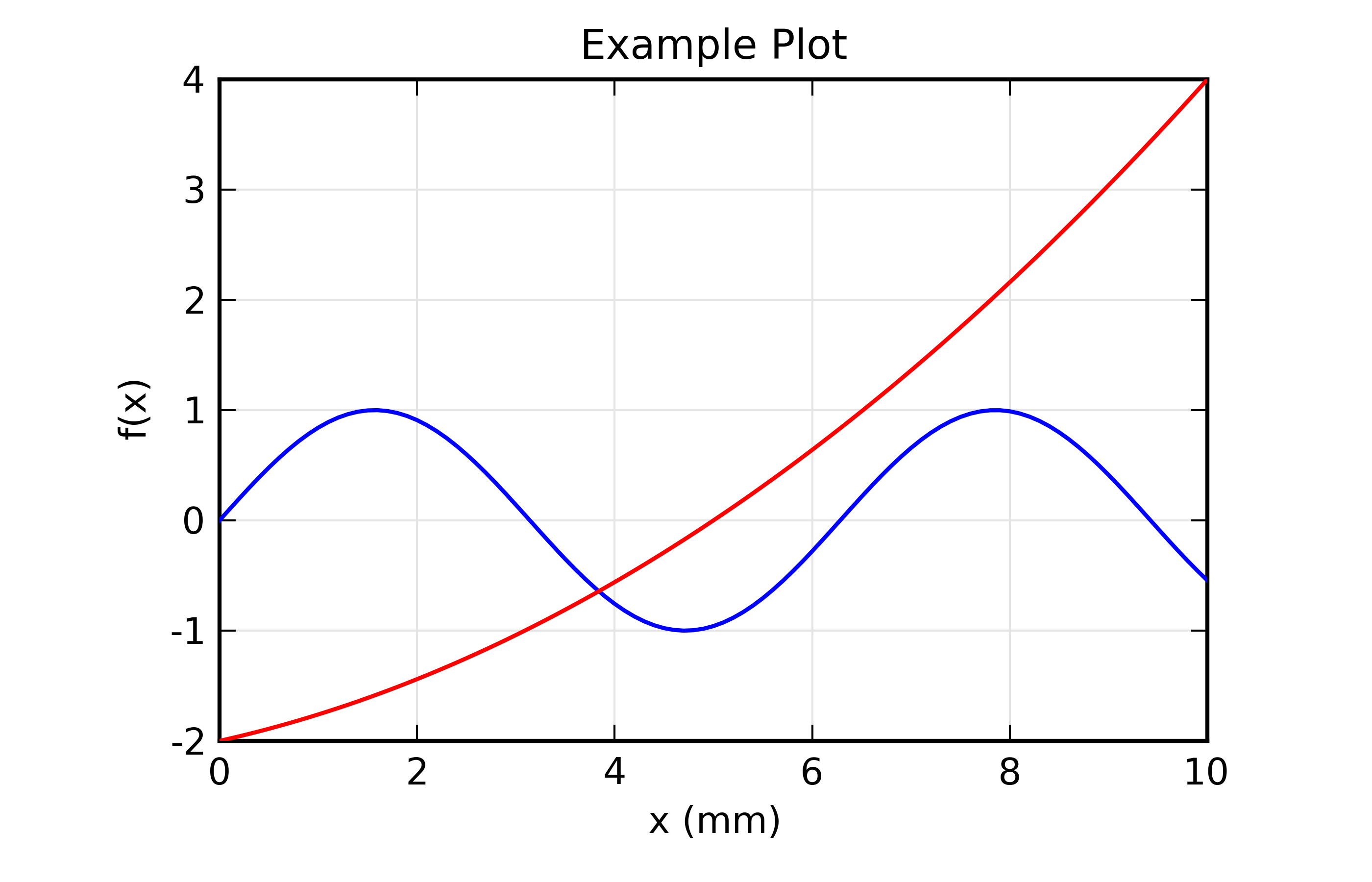Figure 11.1.2.2 A line plot with two curves.

### 11.1.3. Interactive Use of the Plot Windows¶

From the main plot window, you can perform several tasks interactively:

Getting Cursor Position:

From the plot window you can click the left button of your mouse, and see the X, Y coordinates of where you clicked displayed in the status bar at the bottom of the plot window. You can also read the values from the variables _plotter.plot1_x and _plotter.plot1_y, for plot window 1, and _plotter.plot2_x and on for other plot windows.

Zooming in and out:

Left-clicking on the plot window and then dragging the mouse around with the button still pressed will draw a rectangular box around part of the plot window. Releasing the mouse will zoom in on the portion of the plot set by the rectangle. You can zoom in multiple times.

To unzoom, press Ctrl-Z (Apple-Z on Mac OS X), which will go back to the previous zoom rectangle. You can also right-click on the plot, which will bring up a window from which you can zoom out 1 level at a time, or all the way back to fully zoomed out.

Copy to Clipboard:

To copy the plot image (just the main plot image, not all the Window decorations such as menus and status bar) to the sysem clipboard, type Ctrl-C (Apple-C for Mac OS X users). You can then paste this image into other applications such as rich text documents and slide presentations.

Save image to PNG, PDF, or SVG:

To save the plot image to a standard image format, use Ctrl-S (Apple-S for Mac OS X users). This will bring up a ‘save file’ dialog box for writing an image file. The images will be generally high resolution, and should be of sufficiently high quality to be acceptable for publication or public display. Each of the supported image formats – PNG (default), PDF, and SVG – has its strengths and weaknesses. The PNG images use high resolution (600dpi) and anti-aliasing, and are generally higher quality than the SVG images. The PDF images include real font information but does not use anti-aliasing.

Print image:

On many systems, you should be able to print directly from the Plot Window, using Ctrl-P (Apple-P for Mac OS X users). This may not work on all systems, in which case saving an image and printing that should

Configuring the Plot:

From the Plot Window, either Ctrl-K (Apple-K for Mac OS X users) or Options->’Configure Plot’ (or right-click to bring up a popup menu, then select Configure) will bring up the plot configuration window, which looks like this: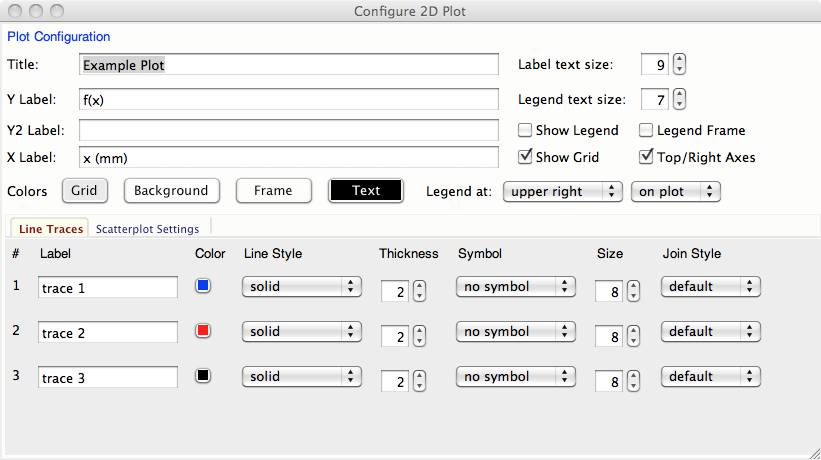Figure 11.1.3.1 Screenshot of the Configuration window for Plots.

From here you can set the titles, axis labels, and styles, colors, symbols, labels, and so on for each of the line traces drawn.

### 11.1.4. Using TeX-like commands for labels and titles¶

The titles and labels for plot elements can be simple strings or use a subset of TeX markup to give fine control over typesetting greek letters, mathematical symbols and formulae. A simple example would be:

plot(k, chi, xlabel = '$k \rm(\AA^{-1})$', ylabel = '$\chi(k)$ ')


The portion of the strings between the dollar signs (‘$’) will be rendered as TeX-like markup, and so render the x and y labels as (for the pedantic, these renderings below may be only approximate): $$k \rm(\AA^{-1})$$ $$\chi(k)$$ An important point here is the use of the backslash character, ‘\’, which you may recall from the tutorial is also used as an escape sequence. Thus some TeX sequences, such as ‘\theta’ may require an additional backslash, so that the ‘\t’ part isn’t rendered as a tab character. More generally, use of raw strings is recommended in this context, so that one uses: plot(k, chi, xlabel = r'$ k \rm(\AA^{-1}) $', ylabel = r'$ \chi(k) $')  Note that this does not actually use TeX (so you don’t need TeX installed), and the rendering is done by the matplotlib library. For further details about using TeX for markup, including a list of symbols, commands to change fonts, and examples, can be found at http://matplotlib.org/users/mathtext.html When using the Plot Configuration window to enter a TeX-like string, the text control box will be given a yellow background color (instead of the normal white color) if there is an error in rendering your TeX string. ## 11.2. Image Display and Contour¶ _plotter.imshow(dat, x=None, y=None, colormap=None, **kws) Parameters • dat – 2-d array of some intensity • x – 1-d array of x values • y – 1-d array of y values • colormap – name of color table to use. display a grey-scale or false-color image from a 2-d array of intensities. _plotter.contour(dat, x=None, y=None, colormap=None, nlevels=None, **kws) Parameters • dat – 2-d array of some intensity • x – 1-d array of x values • y – 1-d array of y values • colormap – name of color table to use. • nlevels – number of contour levels shown display a grey-scale or false-color contour map from a 2-d array of intensities. For both these functions, the x and y arguments are intended to show real world coordinates for the image, not just the array indices. If None, the array indices will be shown. By default, the image will be shown with the origin (pixel [0, 0]) in the lower left corner. The image can be rotated and flipped by the user. The colormap argument is the name of the color map to use to transform intensity to color. The default table is ‘gray’ for a grayscale mapping. Other valid names include ‘coolwarm’, ‘cool’, ‘hot’, ‘jet’, ‘Reds’, ‘Greens’, ‘Blues’, ‘copper’, and a host of others, available From the Image Display Window, you zoom in on regions of the image, rotate the image, change the color table, change the intensity scaling, change the interpolattion algorithm used. You can also toggle between showing an image of continuously varying intensities and a contour map. An example image, generated with ## examples/plotting/doc_image2d.lar def gauss2d(x, y, x0, y0, sx, sy): return outer(exp(-(((y-y0)/float(sy))**2)/2), exp(-(((x-x0)/float(sx))**2)/2)) enddef ny, nx = 350, 400 ix = arange(nx) iy = arange(ny) x = ix / 10. y = -2 + iy / 10.0 dat = 0.2 + (0.05*random.random(size=nx*ny).reshape(ny, nx) + 2.0*gauss2d(ix, iy, 190, 176, 57, 69)) imshow(dat, x=x, y=y, colormap='coolwarm') ## end of examples/plotting/doc_image2d.lar  is shown below: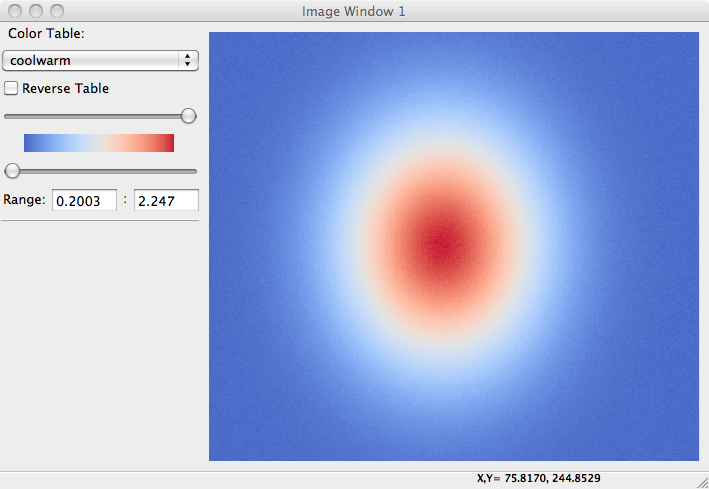Figure 11.2.1 A false-color display of 2 dimensional image data. and as a contour plot, with a different color table: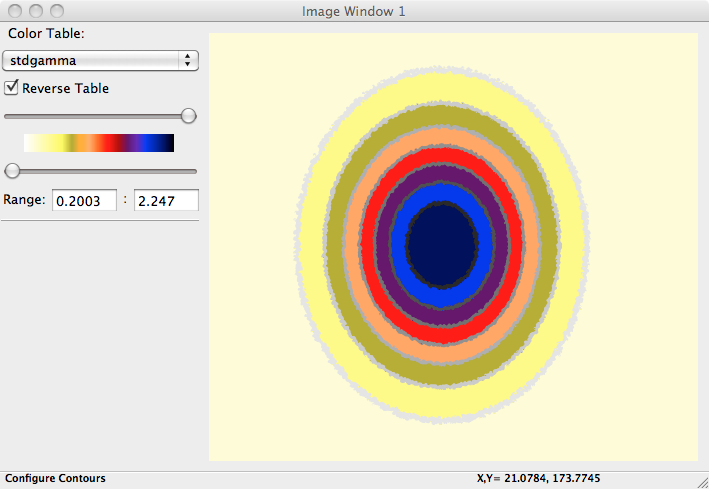Figure 11.2.2 A contour plot of 2 dimensional image data. ## 11.3. Advanced Plotting: using matplotlib¶ So far, this chapter has shown how to make simple plots and images. One of the main goals of Larch is to not only make the simple tasks very simple, but to keep the more difficult tasks possible and accessible. To this end, you can access both the wxPython and matplotlib components of the plots and images to do plotting tasks not covered above. The matplotlib library offers a full range of line and image plotting functionality, as well as some support for 3-dimensioanl plotting. Larch gives you access to the maplotlib API by giving you access to the matplotlib Axes and Canvas for any displayed plot. To get this, you would use get_display() to get the current display window, then access the panel.axes member: larch> x = linspace(0, 10, 101) larch> y = sin(x) larch> plot(x, y) larch> display = get_display(win=1) larch> axes = display.pane.axes  As an example of what you can do with this, here we make a histogram plot from a sampling of a more conitinuous distribution. This uses matplotlib’s hist() function, ## examples/plotting/doc_use_mpl.lar # from scipy.special import gamma title = 'GNXAS-like distribution function' sigma, beta, r0 = 0.12, 0.65, 3.0 q = 4/beta**2 amp = 2 / (sigma*abs(beta)*gamma(q)) # calculate g(r) on a fine r-grid: r = linspace(2.5, 3.5, 201) arg = q + (2*(r-r0)) / (beta*sigma) gr = amp * exp(-arg)*arg**(q-1) # note removal of nan's and impossible values gr[isnan(gr)] = 0 if min(gr)< 0: gr[where(gr<0)] = 0 # standard plot of g(r) newplot(r, gr, grid=False, xlabel=r'$R\,\rm(\AA)$', ylabel=r'$g(R)\$',
title=title, fullbox=False)

# calculate g(r) on a coarse r-grid:
hr   = linspace(2.5, 3.5, 21)
harg = q + (2*(hr-r0)) / (beta*sigma)
hgr  = amp * exp(-harg)*harg**(q-1)
hgr[isnan(hgr)] = 0
if min(hgr)< 0:  hgr[where(hgr<0)] = 0

# get panel, matplotlib axes
panel = get_display().panel
axes  = panel.axes

# plot histogram of coarse-grid data
histo = axes.hist(hr, weights=hgr, bins=len(hr), rwidth=0.25, color='k')
panel.draw()

## end of examples/plotting/doc_use_mpl.lar


which generates a plot that looks like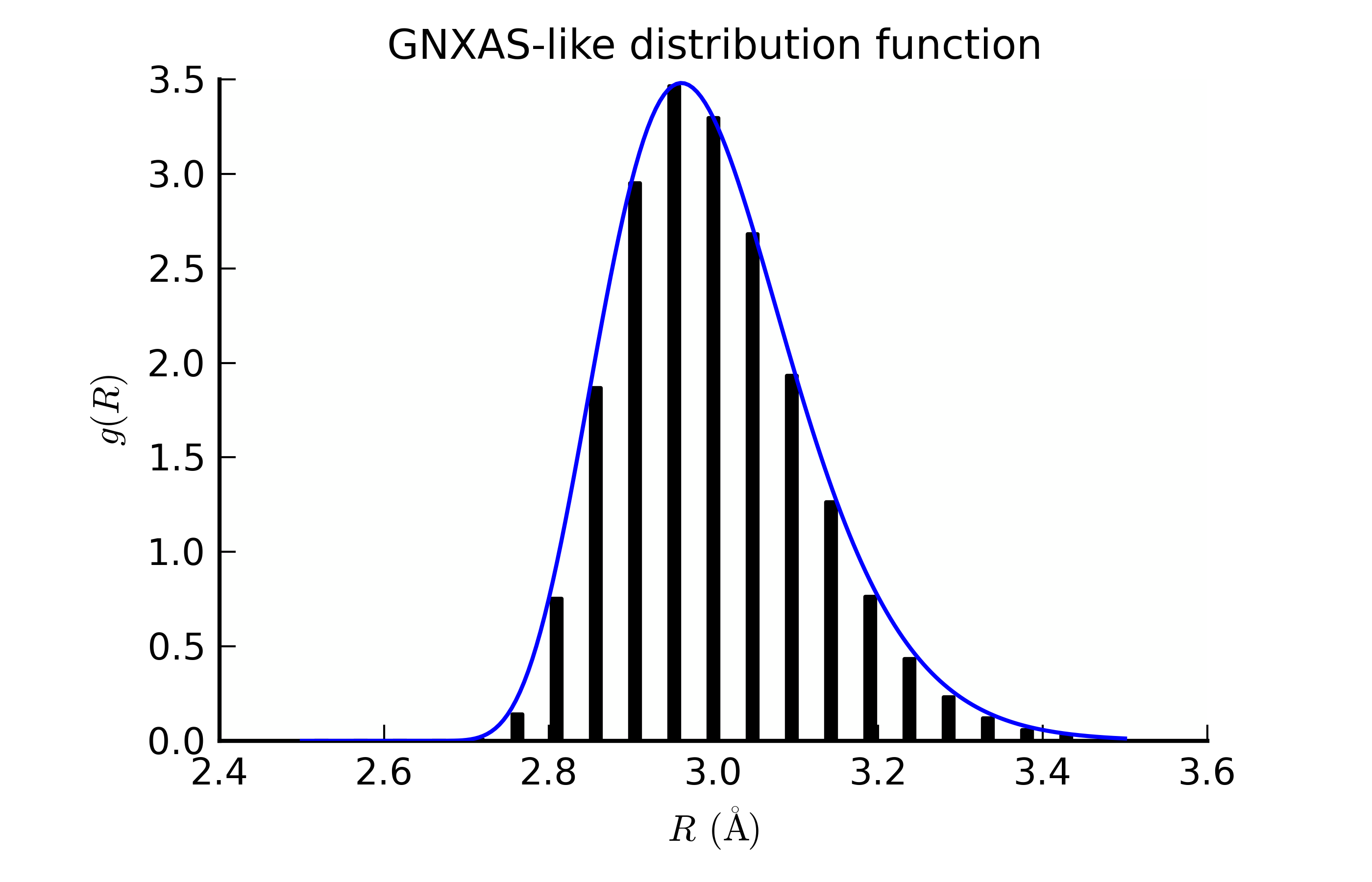Figure 11.3.1 A histogram plot made using matplotlib’s hist() function.